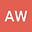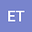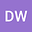Rapid Environmental Quenching of Satellite Dwarf Galaxies in the Local Group
•••• Andrew Wetzel,
• Erik Tollerud,
• Dan Weisz
Andrew Wetzel
Author ProfileErik Tollerud
Author Profile## Abstract

In the Local Group, nearly all of the dwarf galaxies ($$M_{\rm star}\lesssim 10^{9}~{}\mbox{M}_{\odot}$$) that are satellites within $$300~{}\mbox{kpc}$$ (the virial radius) of the Milky Way (MW) and Andromeda (M31) have quiescent star formation and little-to-no cold gas. This contrasts strongly with comparatively isolated dwarf galaxies, which are almost all actively star-forming and gas-rich. This near dichotomy implies a rapid transformation after falling into the halos of the MW or M31. We combine the observed quiescent fractions for satellites of the MW and M31 with the infall times of satellites from the ELVIS suite of cosmological simulations to determine the typical timescales over which environmental processes within the MW/M31 halos remove gas and quench star formation in low-mass satellite galaxies. The quenching timescales for satellites with $$M_{\rm star}<10^{8}~{}\mbox{M}_{\odot}$$ are short, $$\lesssim 2~{}\mbox{Gyr}$$, and decrease at lower $$M_{\rm star}$$. These quenching timescales can be $$1-2~{}\mbox{Gyr}$$ longer if environmental preprocessing in lower-mass groups prior to MW/M31 infall is important. We compare with timescales for more massive satellites from previous works, exploring satellite quenching across the observable range of $$M_{\rm star}=10^{3-11}~{}\mbox{M}_{\odot}$$. The environmental quenching timescale increases rapidly with satellite $$M_{\rm star}$$, peaking at $$\approx 9.5~{}\mbox{Gyr}$$ for $$M_{\rm star}\sim 10^{9}~{}\mbox{M}_{\odot}$$, and rapidly decreases at higher $$M_{\rm star}$$ to less than $$5~{}\mbox{Gyr}$$ at $$M_{\rm star}>5\times 10^{9}~{}\mbox{M}_{\odot}$$. Thus, satellites with $$M_{\rm star}\sim 10^{9}~{}\mbox{M}_{\odot}$$, similar to the Magellanic Clouds, exhibit the longest environmental quenching timescales.